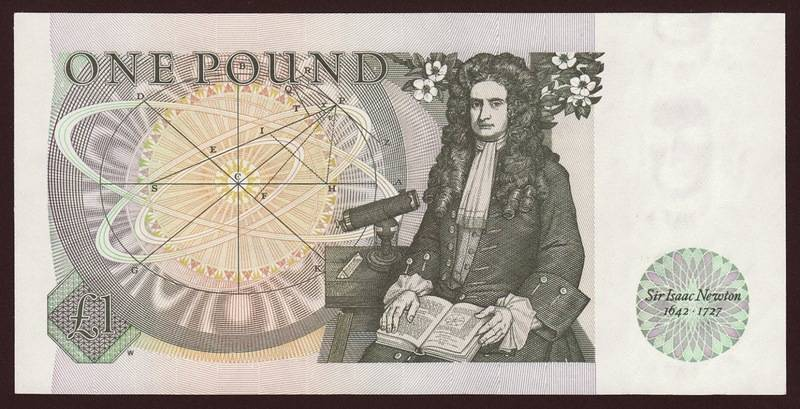# Hooke's law, Bertrand's theorem and closed orbits

• A
Kashmir
Bertrand's Theorem says : the only forces whose bounded orbits imply closed orbits are the Hooke's law and the attractive inverse square force.

I'm looking at the hookes law ##f=-k r## and try to see explicitly that the orbit is indeed closed.

I use the orbit equation ##\frac{d^{2} u}{d \theta^{2}}+u=\frac{-m}{l^{2} u^{2}} f\left(\frac{1}{u}\right)## with the force given as ##f=-k r## ,therefore I get ##\frac{d^{2} u}{d \theta^{2}}+u=+\frac{mk}{l^{2} u 3}## as the equation defining the trajectory.

However neither can I solve this nor can I see that the equation implies a closed orbit.

I'm looking at the hookes law ##f=-k r## and try to see explicitly that the orbit is indeed closed.

Hooke's law gives you harmonic oscillation along each axis in the orbital plane, with a period independent of max. amplitude, thus the same for both axes and equal to the orbital period:
https://en.wikipedia.org/wiki/Harmonic_oscillator

•Kashmir
Kashmir
Hooke's law gives you harmonic oscillation along each axis in the orbital plane, with a period independent of max. amplitude, thus the same for both axes and equal to the orbital period:
https://en.wikipedia.org/wiki/Harmonic_oscillator
Thank you. I got it. We've three SHM along three axis which are periodic, hence a closed orbit.•